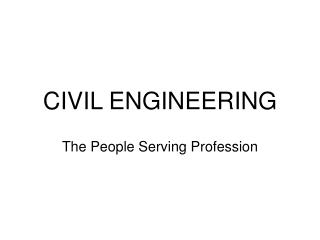# CIVIL ENGINEERING - PowerPoint PPT PresentationDownload PresentationCIVIL ENGINEERING

CIVIL ENGINEERINGDownload Presentation## CIVIL ENGINEERING

- - - - - - - - - - - - - - - - - - - - - - - - - - - E N D - - - - - - - - - - - - - - - - - - - - - - - - - - -
##### Presentation Transcript

1. CIVIL ENGINEERING The People Serving Profession

2. What do Civil Engineers Do?

3. Areas of Study • Structural Engineering • Transportation Engineering • Geotechnical Engineering • Construction Engineering and Management • Water Resources • Environmental

4. Water Resources • Ground water contamination • Remote sensing • Storm water management • Stream channel erosion • Flooding • Urban water resources • Water quality monitoring

5. Environmental • Air and water • Proper management of the environment • Remediation of existing contaminants • Prevention of future contamination • Sustainable resource management At UMBC, Environmental Engineering includes water resources

6. Traditionally • Water distribution networks • Sewage treatment plants • Drinking water treatment plants

7. Now • Detection and modeling fate and transport of contaminants • Restoring environmental quality • Develop industrial processes for ecological preservation and enhance sustainability • http://www3.lehigh.edu/engineering/cee

8. Why Environmental Engineering? • Appeals to girls • Help make the world a better place!

9. Chemistry Review – Acids • Taste sour (vinegar, lemon juice) • Turn blue litmus paper red • Destroy chemical properties of bases • Conduct an electric current • Are corrosive • Can donate a proton or accept electrons to form a covalent bond with a base • pH <7

10. Chemistry Review – Bases • Taste bitter (mustard, cough medicine) • Restore blue color to red litmus paper • Destroy chemical properties of acids • Conduct an electric current • Feel slippery • Can accept a proton or donate electrons to form a covalent bond with an acid • pH >7

11. Strong or Weak? STRONG • Dissociates 100% in solution • The [H+] = concentration of acid • The [OH-] = concentration of base • Has a strong attraction for [H+] or [OH-] WEAK • Dissociate less than 100%

12. Strong Acids • HCl hydrochloric acid • HNO3 nitric acid • H2SO4 sulfuric acid • HBr hydrobromic acid • HI hydroiodic acid • HClO4 perchloric acid

13. Strong Bases • LiOH lithium hydroxide • NaOH sodium hydroxide • KOH potassium hydroxide • RbOH rubidium hydroxide • CsOH cesium hydroxide • *Ca(OH)2 calcium hydroxide • *Sr(OH)2 strontium hydroxide • *Ba(OH)2 barium hydroxide * Completely dissociate in solutions of .01 M or less

14. Salts • The non-water product of an acid – base neutralization Example: HCl + NaOH --> NaCl + H2O

15. pH • -log[H+] • [H+] is in molarity (M = mole/L) • Molarity the number of moles of solute dissolved in a liter of solution (solvent) • For a strong acid, [H+] = [acid] • For a strong base, [OH-] = [base] • pOH = -log[OH-] • pH + pOH = 14

16. pH Examples Strong Acids • 0.10 M solution of HCl pH = - log (0.100) = 1.00 • 1.00 M solution of HBr pH = - log (1.00) = 0.00 • 2.00 M solution of HCl pH = - log (2.00) = -0.30 Strong Bases • 0.10 M solution of NaOH pOH = - log (0.100) = 1.00 pH = 14.00 – 1.00 = 13.00 • 0.050 M solution of KOH pOH = - log (0.050) = 1.30 pH = 14.00 – 1.30 = 12.70

17. Law of Chemical Equilibrium aA + bB cC +dD [C]c[D]d = Ke [A]a[B]b Ke = equilibrium constant a, b, c, d = stochiometric coefficients

18. Equilibrium Constant • Ke measures the extent to which a reaction goes before equilibrium is attained • The larger the Ke: • the larger the quantity of products • The more complete the reaction

19. Calculating the pH • HAc + H2O H3O+ + Ac- (Ac = CH3COOH) • Ka = ( [H3O+ ][Ac-] ) / [HAc] = 1.77 x 10-5 • [H3O+ ] = x and [Ac-] = x because of the one-to-one molar ratio • 1.77 x 10-5 = {(x) (x)} / [HAc] • [HAc] started at 0.100 M ended at (0.100 – x) as HAc molecules dissociated • 1.77 x 10-5 = ((x) (x)) / (0.100-x) solve with the quadratic formula • Or approximate: since x is small, (0.100 – x) appr. equals 0.100 • 1.77 x 10-5 = x2 / 0.100 • x2 = 1.77 x 10-6 • x = 1.33 x 10-3 M = [H3O+] = [H+] • pH = -log(1.33 x 10-3) • pH = 2.87 http://dbhs.wvusd.k12.ca.us/webdocs/AcidBase/AcidBase.html

20. Titration Curves • Show how the pH of a solution changes with the addition of a standard solution of base or acid • Use an acid or base of a known concentration to determine the concentration of an unknown solution • Determine the number of active sites on a solid that can adsorb a contaminant

21. Titration of an Acid

22. Filtration System • Combines carbon filtration with ion exchange and sub-micron filtration • Significantly reduces: • Chlorine • Lead • Synthetic chemicals • Volatile organic compounds • Turbidity • Water-borne parasites

23. Three Step Process • Vacuum Filtration - removal of solids • Granular Activated Carbon - adsorbs molecular organic and inorganic contaminants (metals) • High pressure membrane filtration -removes ions

24. Experiment • Test pH of water before filtration • Base and acid • Titrate solution • Filter solids • Add granular activated carbon (GAC) • Filter out GAC • Repeat titration